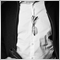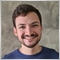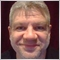# Python API Error Code 10013, Invalid Request21

I open 5-6 positions from the MetaTrader 5 Terminal. Then, via Python-instruction ‘positions_get()‘ I download the open positions and put them in a pandas dataframe. After that I close all open positions with ‘order_send()‘.

The problem is that I can never close all positions.

Sometimes it only closes 2, other times only 3, other times still only 4. The error it reports is always and only this here:

10013 (Invalid Request).

What error is it? It’s the only error where you can’t figure out what kind of error it is.

This is a part of my code:

```# dataPortfolio is the pandas dataframe which contains all open positions downloaded from MetaTrader 5. I have tested it, it is correct.
for index,row in self.dataPortafolio.iterrows(): # we iterate through all open positions
if row['type'] == mt5.ORDER_TYPE_BUY: # if order type is a buy, to close we have to sell
order_type = mt5.ORDER_TYPE_SELL
price = mt5.symbol_info_tick(row['symbol']).bid
else:                   # otherwise, if order type is a sell, to close we have to buy
order_type = mt5.ORDER_TYPE_BUY
price = mt5.symbol_info_tick(row['symbol']).ask
close_request = {
"action": mt5.TRADE_ACTION_DEAL,
"symbol": row['symbol'],
"volume": row['volume'],
"type": order_type,
"position": row['ticket'],
"price": price,
"deviation": 20,
"magic": 234000,
"comment": 'Close trade',
"type_time": mt5.ORDER_TIME_GTC,
"type_filling": mt5.ORDER_FILLING_IOC, # also tried with ORDER_FILLING_RETURN
}
result = mt5.order_send(close_request) # send order to close a position
if result.retcode != mt5.TRADE_RETCODE_DONE:
print("Close Order "+row['symbol']+" failed!!...Error Code: "+str(result.retcode))
else:
print("Order "+row['symbol']+" closed successfully")

```

What’s wrong with my code?1273

Invalid request means, you are requesting something that is not possible.

This is usually caused by values inside the MQLRequest structure, which do not meet the criteria to what you want to do.

An example is an invalid value for SL or TP.
These values need to be treated by NormalizeDouble before sending the request.

Also you should first clear the structure to be all values at NULL.

Hope this helps.602

Ataros:

I open 5-6 positions from the MetaTrader 5 Terminal. Then, via Python-instruction ‘positions_get()‘ I download the open positions and put them in a pandas dataframe. After that I close all open positions with ‘order_send()‘.

The problem is that I can never close all positions.

Sometimes it only closes 2, other times only 3, other times still only 4. The error it reports is always and only this here:

10013 (Invalid Request).

What error is it? It’s the only error where you can’t figure out what kind of error it is.

This is a part of my code:

What’s wrong with my code?

How Dominik Egert has said, it needs to normalize the values before sending them to request.

Also, I usually face this problem, and I notice that the comments sometimes cause it. After normalizing the values, try to change the comment, switching it from the single quote ' to double quote " or even passing an empty string to it just to check if it worked.

https://github.com/Joaopeuko/Mql5-Python-Integration21

Hi Dominik and Joao Paulo, thanks for your suggestions. I also tried to write the comment with double quotes and null string, but I couldn't solve the problem.

These are the changes i made:

```# dataPortfolio is the pandas dataframe which contains all open positions downloaded from MetaTrader 5. I have tested it, it is correct.
for index,row in self.dataPortafolio.iterrows(): # we iterate through all open positions
symbol_info = mt5.symbol_info(row['symbol'])
digits = symbol_info.digits
if row['type'] == mt5.ORDER_TYPE_BUY: # if order type is a buy, to close we have to sell
order_type = mt5.ORDER_TYPE_SELL
price = round(mt5.symbol_info_tick(row['symbol']).bid,digits)
else:                   # otherwise, if order type is a sell, to close we have to buy
order_type = mt5.ORDER_TYPE_BUY
price = round(mt5.symbol_info_tick(row['symbol']).ask,digits)
close_request = {
"action": mt5.TRADE_ACTION_DEAL,
"magic": 234000,
"order": 0,
"symbol": row['symbol'],
"volume": row['volume'],
"price": price,
"stoplimit": 0.0,
"sl": 0.0,
"tp": 0.0,
"deviation": 10,
"type": order_type,
"type_filling": mt5.ORDER_FILLING_IOC, # also tried with ORDER_FILLING_RETURN
"type_time": mt5.ORDER_TIME_GTC,
"expiration": 0,
"comment": "Close Trade",
"position": row['ticket'],
"position_by": 0,
}
result = mt5.order_send(close_request) # send order to close a position
if result.retcode != mt5.TRADE_RETCODE_DONE:
print("Close Order "+row['symbol']+" failed!!...Error Code: "+str(result.retcode))
else:
print("Order "+row['symbol']+" closed successfully")
```1273

Ataros:

Hi Dominik and Joao Paulo, thanks for your suggestions. I also tried to write the comment with double quotes and null string, but I couldn't solve the problem.

These are the changes i made:

Hmm...

you need to round the volume as well.... - I dont knnow if this is the issue.

Additionally you could try ORDER_FILLING_FOK

If all of that does not help, try to print out the structure and see the values given. Post them here. I would like to see.

Could you verify the ticket number does in fact exist?

You could also set deviation to 2* spread... this will ensure, you have nothing standing in between your request and the markets current price.21

```In Input there are these open positions (dataframe pandas 'dataPortfolio'):

ticket                time   type  volume  price_open        sl  price_current  swap  profit   symbol
0  1073335432  8/4/2021 19:20 Gio  Short    0.50    13750.51  14450.51       13741.42   0.0    3.82  [NQ100]
1  1073335441  8/4/2021 19:20 Gio   Long    0.60     4095.91   3695.91        4094.26   0.0   -0.83  [SP500]
2  1073335449  8/4/2021 19:20 Gio  Short    0.40    15202.94  15870.94       15200.25   0.0    1.08  [DAX30]
3  1073335453  8/4/2021 19:20 Gio   Long    0.07     1757.44   1589.44        1757.47   0.0    0.18     GOLD
4  1073335466  8/4/2021 19:20 Gio  Short    0.10    57584.94  81977.94       57888.29   0.0  -25.46   BTCUSD
5  1073335480  8/4/2021 19:20 Gio   Long    1.50       19.31     11.51          19.17   0.0   -1.76   ETCUSD

In Output the order_send function gives me this:

OrderSendResult(retcode=10009, deal=0, order=1073335620, volume=0.5, price=0.0, bid=0.0, ask=0.0, comment='Close Trade', request_id=49, retcode_external=0, request=TradeRequest(action=1, magic=234000, order=0, symbol='[NQ100]', volume=0.5, price=13742.45, stoplimit=0.0, sl=0.0, tp=0.0, deviation=10, type=0, type_filling=1, type_time=0, expiration=0, comment='Close Trade', position=1073335432, position_by=0))
OrderSendResult(retcode=10013, deal=0, order=0, volume=0.0, price=0.0, bid=0.0, ask=0.0, comment='Invalid request', request_id=0, retcode_external=0, request=TradeRequest(action=1, magic=234000, order=0, symbol='[SP500]', volume=0.6, price=4094.91, stoplimit=0.0, sl=0.0, tp=0.0, deviation=10, type=0, type_filling=1, type_time=0, expiration=0, comment='Close Trade', position=1073335441, position_by=0))
OrderSendResult(retcode=10009, deal=0, order=1073335623, volume=0.4, price=0.0, bid=0.0, ask=0.0, comment='Close Trade', request_id=50, retcode_external=0, request=TradeRequest(action=1, magic=234000, order=0, symbol='[DAX30]', volume=0.4, price=15201.26, stoplimit=0.0, sl=0.0, tp=0.0, deviation=10, type=0, type_filling=1, type_time=0, expiration=0, comment='Close Trade', position=1073335449, position_by=0))
OrderSendResult(retcode=10013, deal=0, order=0, volume=0.0, price=0.0, bid=0.0, ask=0.0, comment='Invalid request', request_id=0, retcode_external=0, request=TradeRequest(action=1, magic=234000, order=0, symbol='GOLD', volume=0.07, price=1757.46, stoplimit=0.0, sl=0.0, tp=0.0, deviation=10, type=0, type_filling=1, type_time=0, expiration=0, comment='Close Trade', position=1073335453, position_by=0))
OrderSendResult(retcode=10009, deal=0, order=1073335625, volume=0.1, price=0.0, bid=0.0, ask=0.0, comment='Close Trade', request_id=51, retcode_external=0, request=TradeRequest(action=1, magic=234000, order=0, symbol='BTCUSD', volume=0.1, price=57890.06, stoplimit=0.0, sl=0.0, tp=0.0, deviation=10, type=0, type_filling=1, type_time=0, expiration=0, comment='Close Trade', position=1073335466, position_by=0))
OrderSendResult(retcode=10013, deal=0, order=0, volume=0.0, price=0.0, bid=0.0, ask=0.0, comment='Invalid request', request_id=0, retcode_external=0, request=TradeRequest(action=1, magic=234000, order=0, symbol='ETCUSD', volume=1.5, price=19.35, stoplimit=0.0, sl=0.0, tp=0.0, deviation=10, type=0, type_filling=1, type_time=0, expiration=0, comment='Close Trade', position=1073335480, position_by=0))
```
As you can see 3 Orders have been accepted (NQ100, DAX30, BTCUSD) and 3 rejected (SP500, GOLD, ETCUSD).1273

Yes.

Here seems to be the issue. Your code cannot close long positions.

I suspect the issue is type. order_type is assigning a wrong value.

It looks like you are trying to close a buy with a buy order.21

You're right Dominik, there was a 'short' circuit in my brain, and I can't explain why.

In my mind I was convinced to make the comparison with the values 0 and 1, but in reality I had put the strings 'Long' and 'Short' in the dataframe, this for my convenience.

Unforgivable mistake!!

Okay, thanks again.41521

Ataros:

You're right Dominik, there was a 'short' circuit in my brain, and I can't explain why.

In my mind I was convinced to make the comparison with the values 0 and 1, but in reality I had put the strings 'Long' and 'Short' in the dataframe, this for my convenience.

Unforgivable mistake!!

Okay, thanks again.

There is no such thing as an "unforgivable mistake". We are all humans, mistakes and errors are how we are learning. ;-)1273

So true.